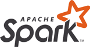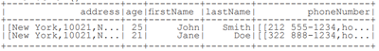HomeCheat sheetsBig Data

# PySpark Cheat Sheet: Spark DataFrames in Python

This PySpark SQL cheat sheet is your handy companion to Apache Spark DataFrames in Python and includes code samples.
Jul 2021  · 5 min read

You'll probably already know about Apache Spark, the fast, general and open-source engine for big data processing; It has built-in modules for streaming, SQL, machine learning and graph processing. Spark allows you to speed analytic applications up to 100 times faster compared to other technologies on the market today. Interfacing Spark with Python is easy with PySpark: this Spark Python API exposes the Spark programming model to Python.

The PySpark Basics cheat sheet already showed you how to work with the most basic building blocks, RDDs.

Now, it's time to tackle the Spark SQL module, which is meant for structured data processing, and the DataFrame API, which is not only available in Python, but also in Scala, Java, and R. If you want to know more about the differences between RDDs, DataFrames, and DataSets, consider taking a look at Apache Spark in Python: Beginner's Guide.

Without further ado, here's the cheat sheet:Have this cheat sheet at your fingertips

This PySpark SQL cheat sheet covers the basics of working with the Apache Spark DataFrames in Python: from initializing the SparkSession to creating DataFrames, inspecting the data, handling duplicate values, querying, adding, updating or removing columns, grouping, filtering or sorting data. You'll also see that this cheat sheet also on how to run SQL Queries programmatically, how to save your data to parquet and JSON files, and how to stop your SparkSession.

Make sure to check out our other Python cheat sheets for data science, which cover topics such as Python basicsNumpyPandasPandas Data Wrangling and much more!Spark SGlL is Apache Spark's module for working with structured data.

## Initializing SparkSession

A SparkSession can be used create DataFrame, register DataFrame as tables, execute SGL over tables, cache tables, and read parquet files.

``>>> from pyspark.sql import SparkSession>>> spark a SparkSession \     .builder\     .appName("Python Spark SQL basic example") \     .config("spark.some.config.option", "some-value") \     .getOrCreate()``

## Creating DataFrames

### Fromm RDDs

``>>> from pyspark.sql.types import*``

Infer Schema

``>>> sc = spark.sparkContext>>> lines = sc.textFile(''people.txt'')>>> parts = lines.map(lambda l: l.split(","))>>> people = parts.map(lambda p: Row(nameap,ageaint(p[l])))>>> peopledf = spark.createDataFrame(people)``

Specify Schema

``>>> people = parts.map(lambda p: Row(name=p,               age=int(p.strip())))>>>  schemaString = "name age">>> fields = [StructField(field_name, StringType(), True) for field_name in schemaString.split()]>>> schema = StructType(fields)>>> spark.createDataFrame(people, schema).show()``

From Spark Data Sources
JSON

``>>>  df = spark.read.json("customer.json")>>> df.show()````>>>  df2 = spark.read.load("people.json", format="json")``

Parquet files

``>>> df3 = spark.read.load("users.parquet")``

TXT files

``>>> df4 = spark.read.text("people.txt")``

## Filter

``#Filter entries of age, only keep those records of which the values are >24>>> df.filter(df["age"]>24).show()``

## Duplicate Values

``>>> df = df.dropDuplicates()``

## Queries

``>>> from pyspark.sql import functions as F``

Select

``>>> df.select("firstName").show() #Show all entries in firstName column>>> df.select("firstName","lastName") \      .show()>>> df.select("firstName", #Show all entries in firstName, age and type              "age",              explode("phoneNumber") \              .alias("contactInfo")) \      .select("contactInfo.type",              "firstName",              "age") \      .show()>>> df.select(df["firstName"],df["age"]+ 1) #Show all entries in firstName and age, .show() add 1 to the entries of age>>> df.select(df['age'] > 24).show() #Show all entries where age >24``

When

``>>> df.select("firstName", #Show firstName and 0 or 1 depending on age >30               F.when(df.age > 30, 1) \              .otherwise(0)) \      .show()>>> df[df.firstName.isin("Jane","Boris")] #Show firstName if in the given options.collect()``

Like

``>>> df.select("firstName", #Show firstName, and lastName is TRUE if lastName is like Smith              df.lastName.like("Smith")) \     .show()``

Startswith - Endswith

``>>> df.select("firstName", #Show firstName, and TRUE if lastName starts with Sm              df.lastName \                .startswith("Sm")) \      .show()>>> df.select(df.lastName.endswith("th"))\ #Show last names ending in th      .show()``

Substring

``>>> df.select(df.firstName.substr(1, 3) \ #Return substrings of firstName                          .alias("name")) \        .collect()``

Between

``>>> df.select(df.age.between(22, 24)) \ #Show age: values are TRUE if between 22 and 24          .show()``

## Add, Update & Remove Columns

`` >>> df = df.withColumn('city',df.address.city) \            .withColumn('postalCode',df.address.postalCode) \            .withColumn('state',df.address.state) \            .withColumn('streetAddress',df.address.streetAddress) \            .withColumn('telePhoneNumber', explode(df.phoneNumber.number)) \            .withColumn('telePhoneType', explode(df.phoneNumber.type)) ``

Updating Columns

``>>> df = df.withColumnRenamed('telePhoneNumber', 'phoneNumber')``

Removing Columns

``  >>> df = df.drop("address", "phoneNumber") >>> df = df.drop(df.address).drop(df.phoneNumber) ``

## Missing & Replacing Values

``>>> df.na.fill(50).show() #Replace null values >>> df.na.drop().show() #Return new df omitting rows with null values >>> df.na \ #Return new df replacing one value with another       .replace(10, 20) \       .show()``

## GroupBy

``>>> df.groupBy("age")\ #Group by age, count the members in the groups      .count() \      .show()``

## Sort

``>>> peopledf.sort(peopledf.age.desc()).collect()>>> df.sort("age", ascending=False).collect()>>> df.orderBy(["age","city"],ascending=[0,1])\     .collect()``

## Repartitioning

``>>> df.repartition(10)\ #df with 10 partitions      .rdd \      .getNumPartitions()>>> df.coalesce(1).rdd.getNumPartitions() #df with 1 partition``

## Running Queries Programmatically

Registering DataFrames as Views

``>>> peopledf.createGlobalTempView("people")>>> df.createTempView("customer")>>> df.createOrReplaceTempView("customer")``

Query Views

``>>> df5 = spark.sql("SELECT * FROM customer").show()>>> peopledf2 = spark.sql("SELECT * FROM global_temp.people")\               .show()``

## Inspect Data

``>>> df.dtypes #Return df column names and data types>>> df.show() #Display the content of df>>> df.head() #Return first n rows>>> df.first() #Return first row>>> df.take(2) #Return the first n rows >>> df.schema Return the schema of df>>> df.describe().show() #Compute summary statistics >>> df.columns Return the columns of df>>> df.count() #Count the number of rows in df>>> df.distinct().count() #Count the number of distinct rows in df>>> df.printSchema() #Print the schema of df>>> df.explain() #Print the (logical and physical) plans``

## Output

Data Structures

`` >>> rdd1 = df.rdd #Convert df into an RDD >>> df.toJSON().first() #Convert df into a RDD of string >>> df.toPandas() #Return the contents of df as Pandas DataFrame``

Write & Save to Files

``>>> df.select("firstName", "city")\       .write \       .save("nameAndCity.parquet") >>> df.select("firstName", "age") \       .write \       .save("namesAndAges.json",format="json")``

## Stopping SparkSession

``>>> spark.stop()``

Introduction to PySpark

Big Data Fundamentals with PySpark

Cleaning Data with PySpark

Feature Engineering with PySpark

Machine Learning with PySpark

Building Recommendation Engines with PySpark

Related

### Pandas 2.0: What’s New and Top Tips

Dive into pandas 2.0, the latest update of the essential data analysis library, with new features like PyArrow integration, nullable data types, and non-nanosecond datetime resolution for better performance and efficiency.

Moez Ali

9 min

### GeoPandas Tutorial: An Introduction to Geospatial Analysis

Get started with GeoPandas, one of the most popular Python libraries for geospatial analysis.

### Python Seaborn Line Plot Tutorial: Create Data Visualizations

Discover how to use Seaborn, a popular Python data visualization library, to create and customize line plots in Python.

### Python Plotly Express Tutorial: Unlock Beautiful Visualizations

Learn how to create highly interactive and visually appealing charts with Python Plotly Express.Bekhruz Tuychiev

10 min

### An Introduction to Python T-Tests

Learn how to perform t-tests in Python with this tutorial. Understand the different types of t-tests - one-sample test, two-sample test, paired t-test, and Welch’s test, and when to use them.Vidhi Chugh

13 min

### Matplotlib time series line plot

This tutorial explores how to create and customize time series line plots in matplotlib.
See MoreSee More